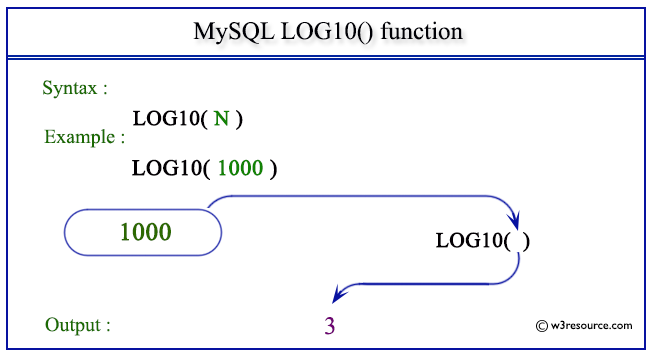# MySQL LOG10() function

## LOG10() function

MySQL LOG10() returns the natural logarithm of a number to the base 10. The equivalent expression of LOG10(N) is LOG(10,N).

Syntax:

```LOG10(N);
```

Where N is a number

Syntax Diagram:MySQL Version: 5.6

Pictorial presentation of MySQL LOG10() functionExample of MySQL LOG10() function

Code:

``````SELECT LOG10(1000);
```
```

Explanation

The above MySQL statement returns the natural logarithm of 1000 as specified in the argument to the base 10.

Sample Output:

```mysql> SELECT LOG10(1000);
+-------------+
| LOG10(1000) |
+-------------+
|           3 |
+-------------+
1 row in set (0.00 sec)
```

All Mathematical FunctionsPrevious: LOG2()
Next: MOD()

﻿